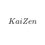# Latex for Vertical Math Operations

How can I do math operations in Latex that goes down vertically? Instead of $1+2=3$, how can I do the one where it goes down by solving?Note by Kaizen Cyrus
4 months, 1 week ago

This discussion board is a place to discuss our Daily Challenges and the math and science related to those challenges. Explanations are more than just a solution — they should explain the steps and thinking strategies that you used to obtain the solution. Comments should further the discussion of math and science.

When posting on Brilliant:

• Use the emojis to react to an explanation, whether you're congratulating a job well done , or just really confused .
• Ask specific questions about the challenge or the steps in somebody's explanation. Well-posed questions can add a lot to the discussion, but posting "I don't understand!" doesn't help anyone.
• Try to contribute something new to the discussion, whether it is an extension, generalization or other idea related to the challenge.

MarkdownAppears as
*italics* or _italics_ italics
**bold** or __bold__ bold
- bulleted- list
• bulleted
• list
1. numbered2. list
1. numbered
2. list
Note: you must add a full line of space before and after lists for them to show up correctly
paragraph 1paragraph 2

paragraph 1

paragraph 2

[example link](https://brilliant.org)example link
> This is a quote
This is a quote
    # I indented these lines
# 4 spaces, and now they show
# up as a code block.

print "hello world"
# I indented these lines
# 4 spaces, and now they show
# up as a code block.

print "hello world"
MathAppears as
Remember to wrap math in $$ ... $$ or $ ... $ to ensure proper formatting.
2 \times 3 $2 \times 3$
2^{34} $2^{34}$
a_{i-1} $a_{i-1}$
\frac{2}{3} $\frac{2}{3}$
\sqrt{2} $\sqrt{2}$
\sum_{i=1}^3 $\sum_{i=1}^3$
\sin \theta $\sin \theta$
\boxed{123} $\boxed{123}$

Sort by:

$\begin{array}{c}&1& \\ +& 2& \\ \hline &3& \end{array}$

- 4 months ago

How can I do that using Latex?

- 4 months ago

\begin{array}&1& \ +& 2& \ \hline \ &3& \end{array}

- 4 months ago

Thank you so much.

- 4 months ago

Sure, you're welcome!

- 4 months ago

Is there a way to somehow make the horizontal line to be directly at the top of the sum? There's so much space between them.

- 4 months ago

Something that looks like now? Then remove the the the extra line of \

- 4 months ago

Got it. Very appreciated. Thanks for responding.

- 4 months ago

You're welcome. Ask if you have any more queries! Good luck!

- 4 months ago

May I also ask how to do the division operation?

- 4 months ago

Sure, you can use \frac{1}{2} with {1} brackets for numerator and {2} denominator respectively. When wrapped in latex, this will result in something like this $\frac{1}{2}$

- 4 months ago

Is it possible as the same manner as your first comment? As in the division operation is going down vertically like it is being solved in an elementary school.

- 4 months ago

You can use online $\mathbf{LaTeX}$ editors and the Chrome Easy Math Editor as well as the Formatting Guide to assist you in mathematical operations. Hope this helps :)

- 3 months, 1 week ago